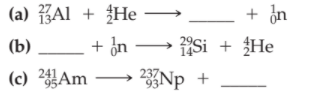×
Get Full Access to Introductory Chemistry - 5 Edition - Chapter 17 - Problem 88p
Get Full Access to Introductory Chemistry - 5 Edition - Chapter 17 - Problem 88p

×

# Solved: Complete each nuclear equation.(a) (b) (c)ISBN: 9780321910295 34

## Solution for problem 88P Chapter 17

Introductory Chemistry | 5th Edition

• Textbook Solutions
• 2901 Step-by-step solutions solved by professors and subject experts
• Get 24/7 help from StudySoup virtual teaching assistantsIntroductory Chemistry | 5th Edition

4 5 1 357 Reviews
16
1
Problem 88P

Complete each nuclear equation.Step-by-Step Solution:
Step 1 of 3

A nuclear equation represents changes that occur during nuclear process such as radioactivity .

For a nuclear equation to be balanced ,the sum of atomic numbers on both the sides of the equation must be equal and the sum of the mass numbers on both sides of the equation must also be equal

a)2313 Al + 42 He1530 P+ 10 n

b) 3216Sc)241Am95 → 237Np93 + 4He2

Nuclear reactions need to have the sum of protons and neutrons the same on both sides of the equation. The number of protons must also be consistent on both sides of the reaction.

Alpha decay occurs when the nucleus of an atom spontaneously ejects an alpha particle. The alpha particle is the same as a helium nucleus with 2 protons and 2 neutrons. This means the number of protons in the nucleus is reduced by 2 and the total number of nucleons is reduced by 4.

241Am95 → ZXA + 4He2

A = number of protons = 95 - 2 = 93

X = the element with atomic number = 93

According to the periodic table, X = neptunium or Np.

The mass number is reduced by 4.

Z = 241 - 4 = 237

Substitute these values into the reaction:

241Am95 → 237Np93 + 4He2

Step 2 of 3

Step 3 of 3

##### ISBN: 9780321910295

Unlock Textbook Solution